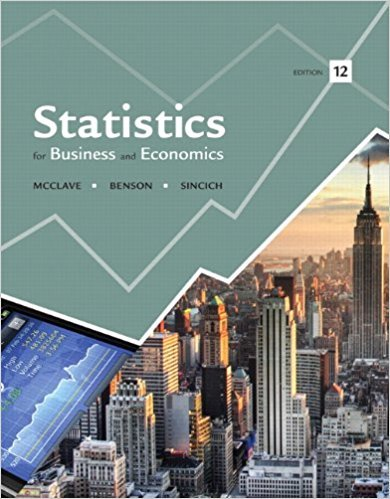×
Log in to StudySoup
Get Full Access to Statistics For Business And Economics - 12 Edition - Chapter 4 - Problem 108e
Join StudySoup for FREE
Get Full Access to Statistics For Business And Economics - 12 Edition - Chapter 4 - Problem 108e

Already have an account? Login here
×
Reset your password

# Ambulance response time. Ambulance response time isISBN: 9780321826237 51

## Solution for problem 108E Chapter 4

Statistics for Business and Economics | 12th Edition

• Textbook Solutions
• 2901 Step-by-step solutions solved by professors and subject experts
• Get 24/7 help from StudySoup virtual teaching assistantsStatistics for Business and Economics | 12th Edition

4 5 1 362 Reviews
23
1
Problem 108E

Problem 108E

Ambulance response time. Ambulance response time is measured as the time (in minutes) between the initial call to emergency medical services (EMS) and when the patient is reached by ambulance. Geographical Analysis (Vol. 41, 2009) investigated the characteristics of ambulance response time for EMS calls in Edmonton, Alberta. For a particular EMS station (call it Station A), ambulance response time is known to be normally distributed with µ= 7.5 minutes and σ= 2.5 minutes.

a. Regulations require that 90% of all emergency calls should be reached in 9 minutes or less. Are the regulations met at EMS Station A? Explain.

b. A randomly selected EMS call in Edmonton has an ambulance response time of 2 minutes. Is it likely that this call was serviced by Station A? Explain.

Step-by-Step Solution:

Solution :

Step 1 of 2:

Given for a particular EMS station ambulance response time is known to be normally distributed with mean= 7.5 per minute and standard deviation=2.5 minute.

Our goal is :

a). We need to find are the regulation met at EMS station and explain.

b). We need to find is it likely that this call was serviced by station A and explain.

a).

Given regulations require that 90% of all emergency call be reached in 9 minute or less.

Now we have to find are the regulation met at EMS station.

The formula for the z score is

z =We know thatand.

Here x = 9.

Then,

z =z =z = 0.6

Therefore, z=0.6.

Then the probability of x is less than or equal to 9 is

P(x9) = P(z0.6)

From area under the normal table.

P(x9) = 0.7257

Hence this probability is less than 0.90, then the regulations are not being met at EMS station A.

b).

Given a randomly selected EMS call in Edmonton has an ambulance response time of 2 minute.

Now we have to find is it likely that this call was serviced by station A.

The formula for the z score is

z =We know thatand.

Here x = 2.

Then,

z =z =z = -2.2

Therefore, z=-2.2.

Then the probability of x is less than or equal to 2 is

P(x2) = P(z-2.2)

From area under the normal table.

P(x2) = 0.0179

Hence this probability is so small, so it would be very unlikely that the call was service by Station A.

Step 2 of 1

##### ISBN: 9780321826237

The answer to “Ambulance response time. Ambulance response time is measured as the time (in minutes) between the initial call to emergency medical services (EMS) and when the patient is reached by ambulance. Geographical Analysis (Vol. 41, 2009) investigated the characteristics of ambulance response time for EMS calls in Edmonton, Alberta. For a particular EMS station (call it Station A), ambulance response time is known to be normally distributed with µ= 7.5 minutes and ?= 2.5 minutes.a. Regulations require that 90% of all emergency calls should be reached in 9 minutes or less. Are the regulations met at EMS Station A? Explain.________________b. A randomly selected EMS call in Edmonton has an ambulance response time of 2 minutes. Is it likely that this call was serviced by Station A? Explain.” is broken down into a number of easy to follow steps, and 126 words. The full step-by-step solution to problem: 108E from chapter: 4 was answered by , our top Business solution expert on 07/21/17, 05:42AM. Since the solution to 108E from 4 chapter was answered, more than 605 students have viewed the full step-by-step answer. This textbook survival guide was created for the textbook: Statistics for Business and Economics , edition: 12. This full solution covers the following key subjects: ambulance, EMS, response, minutes, Call. This expansive textbook survival guide covers 15 chapters, and 1631 solutions. Statistics for Business and Economics was written by and is associated to the ISBN: 9780321826237.

Unlock Textbook Solution

Enter your email below to unlock your verified solution to:

Ambulance response time. Ambulance response time is